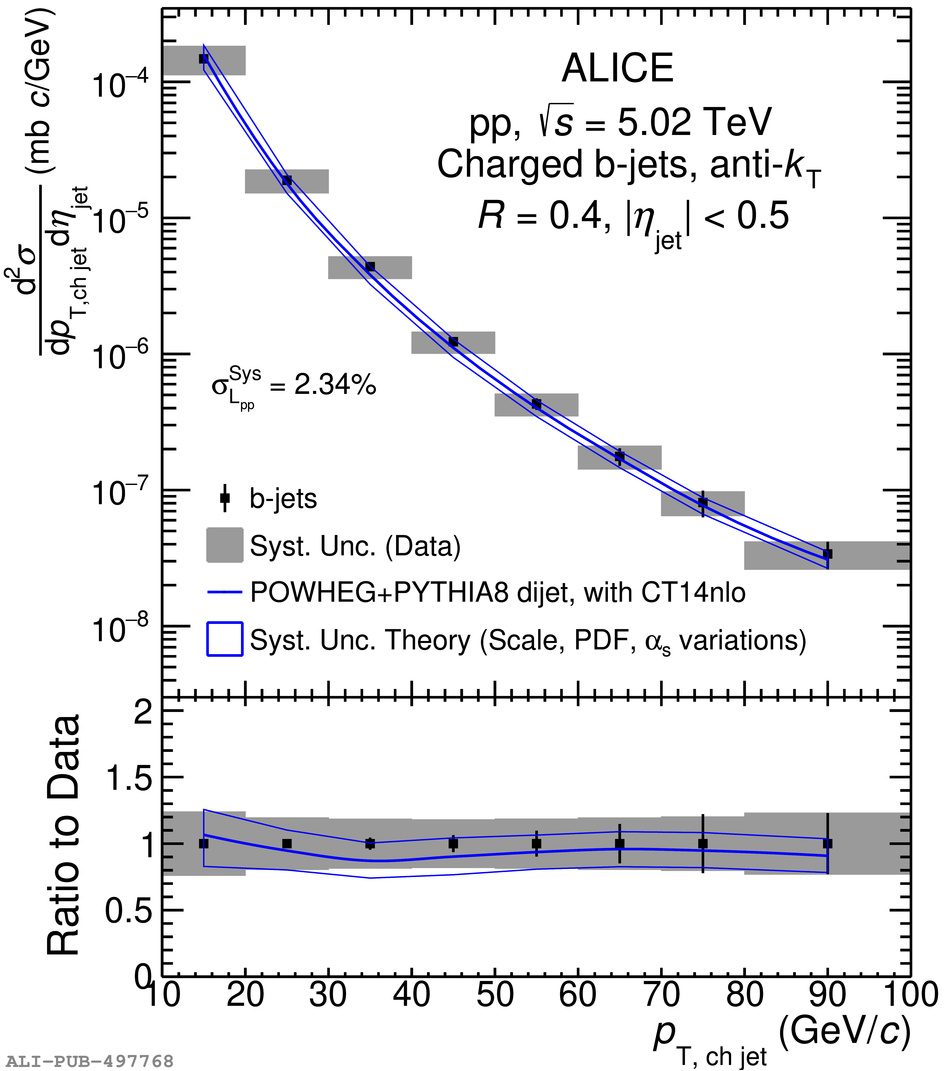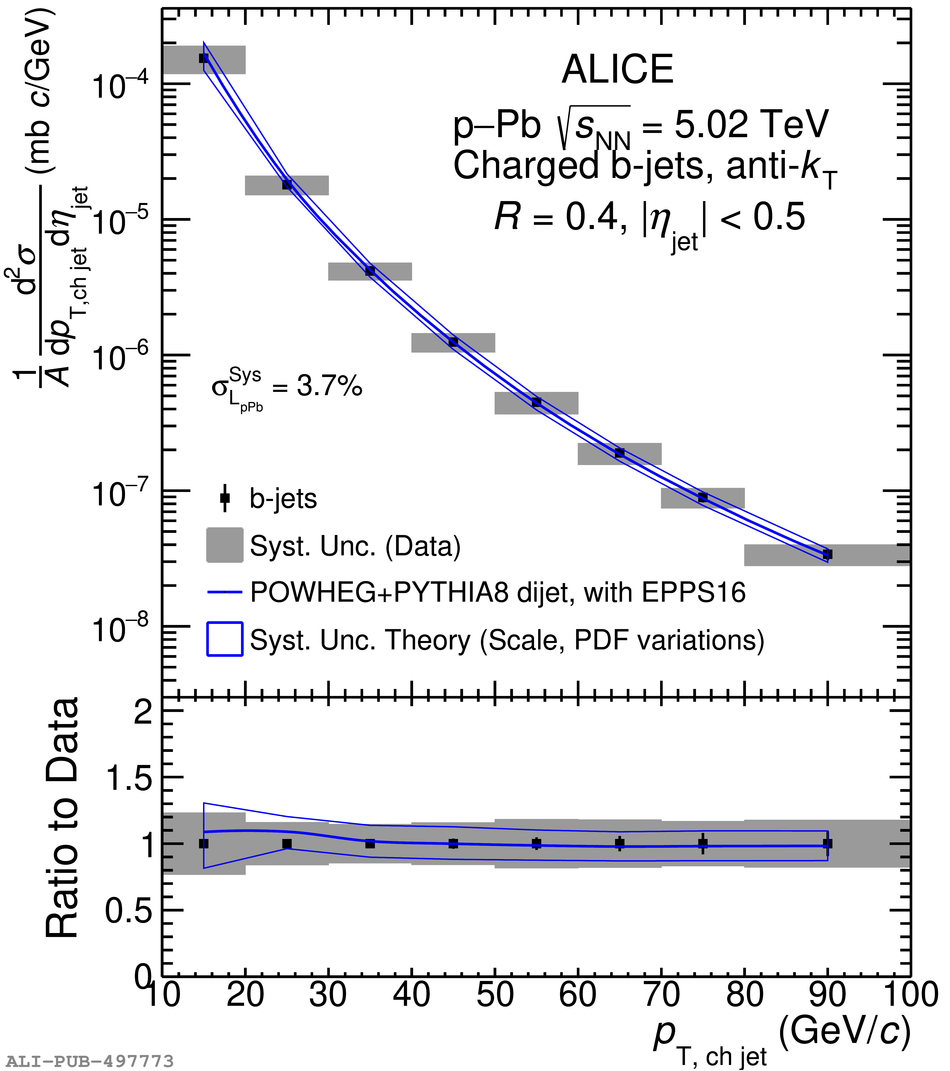# Figure 12

 Top panels: The combined differential production cross section of charged-particle anti-$k_{\rm T}$ $R=0.4$ b jets measured in \pp (left) and \pPb (right) collisions at $\snn=5.02$\,TeV. The data are compared with a NLO pQCD prediction by the POWHEG dijet tune with PYTHIA~8 fragmentation~. Systematic and statistical uncertainties are shown as boxes and error bars, respectively. The additional common normalization uncertainty due to luminosity, $\sigma_{\mathcal{L}}^{\rm Sys}$, is quoted separately. Bottom panels: Ratio of the theory calculations to the data.